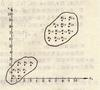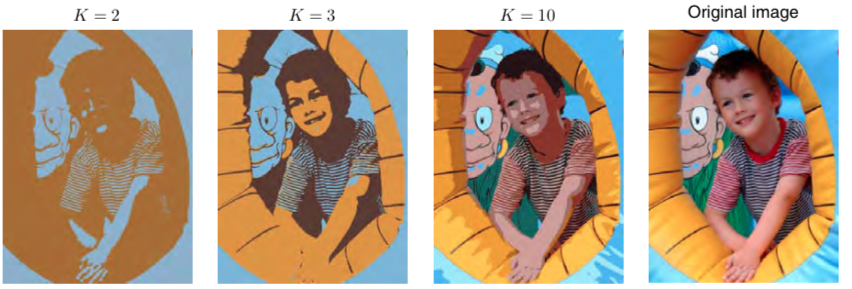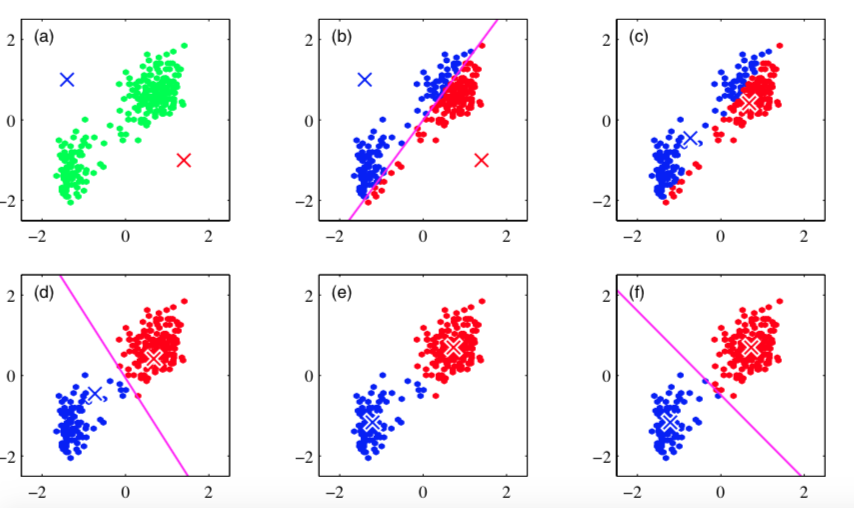kmeans 订阅
k均值聚类算法（k-means clustering algorithm）是一种迭代求解的聚类分析算法，其步骤是，预将数据分为K组，则随机选取K个对象作为初始的聚类中心，然后计算每个对象与各个种子聚类中心之间的距离，把每个对象分配给距离它最近的聚类中心。聚类中心以及分配给它们的对象就代表一个聚类。每分配一个样本，聚类的聚类中心会根据聚类中现有的对象被重新计算。这个过程将不断重复直到满足某个终止条件。终止条件可以是没有（或最小数目）对象被重新分配给不同的聚类，没有（或最小数目）聚类中心再发生变化，误差平方和局部最小。 展开全文k均值聚类算法（k-means clustering algorithm）是一种迭代求解的聚类分析算法，其步骤是，预将数据分为K组，则随机选取K个对象作为初始的聚类中心，然后计算每个对象与各个种子聚类中心之间的距离，把每个对象分配给距离它最近的聚类中心。聚类中心以及分配给它们的对象就代表一个聚类。每分配一个样本，聚类的聚类中心会根据聚类中现有的对象被重新计算。这个过程将不断重复直到满足某个终止条件。终止条件可以是没有（或最小数目）对象被重新分配给不同的聚类，没有（或最小数目）聚类中心再发生变化，误差平方和局部最小。

1967年

James MacQueen

K均值聚类算法

k-means clustering algorithm
K均值聚类算法定义

• ## Kmeans

千次阅读 2015-07-21 11:15:20
Kmeans 实现代码；测试数据以及测试结果分析稍后
/**

*
*
*
*
* */
import java.io.FileNotFoundException;
import java.io.FileWriter;
import java.io.IOException;
import java.util.ArrayList;
import java.util.HashMap;
import java.util.HashSet;
import java.util.List;
import java.util.Map;
import java.util.Random;
import java.util.Set;

public class kMeans {

private static int k;
private String dataFilePath;
private int featureCount;
private static Double SSE = Double.MAX_VALUE;
List<Double[]> srcData = new ArrayList<Double[]>();
List<String> correctClass =  new ArrayList<String>();
static Double[][] kCores ;
Map<Integer,List<Double[]>> Cdata = new HashMap<Integer,List<Double[]>>();

public kMeans(int k ,int featureCount ,String dataFilePath) throws IOException{
this.k = k;
this.featureCount = featureCount;
this.dataFilePath = dataFilePath;
kCores = new Double[k][featureCount+1];
initSrcData();
initKcoresByRandomFunction();
Cluster();
}

public kMeans(int k , int featureCount ,String dataFilePath,double SEthreadhold) throws IOException{
this(k ,featureCount,dataFilePath);
}

void initSrcData(){
int count = 0;
try {
String s;

Double[] srcDataTep = new Double[featureCount+1];
srcDataTep = (double)(++count);
String tep[] = s.split(",");
for(int i=1;i<tep.length;i++)
srcDataTep[i] = Double.valueOf(tep[i]);
}
br.close();
} catch (FileNotFoundException e) {
// TODO Auto-generated catch block
System.out.println("srcData FilePath is not accessable!");
e.printStackTrace();
} catch (IOException e) {
// TODO Auto-generated catch block
e.printStackTrace();
}
}

void initKcoresByRandomFunction(){

Set<Integer> seeds = new HashSet<Integer>();
Random rand = new Random();
int i = 0;
while(i<k){
int index = rand.nextInt(srcData.size()-1);
while(seeds.contains(index)){
index = rand.nextInt(srcData.size()-1);
}

for(int j=1;j<featureCount+1;j++){
kCores[i][j] = srcData.get(index)[j];
}
i++;
}
}

boolean clusterOnce() throws IOException{

Cdata.clear();
System.out.println(srcData.size());
for(Double[] s:srcData){
int index = findNearest(s);
//  System.out.println(index);
List<Double[]> tep;
if(Cdata.containsKey(index)){
tep = Cdata.get(index);
}
else{
tep = new ArrayList<Double[]>();
}
Cdata.put(index, tep);
}
newCores();

if(newSSE() == SSE)
return false;
else{
SSE = newSSE();
return true;
}

}

void Cluster() throws IOException{
boolean flag = clusterOnce();
flag = clusterOnce();
System.out.println(SSE);
}
writeResult2File();
}

int findNearest(Double[] s){
double DistanceTep = Double.MAX_VALUE;
int index = 0;
for(int i=0;i<k;i++){
if(Distance(s,kCores[i])<DistanceTep){

index = i;
DistanceTep = Distance(s,kCores[i]);
}

}
return index;
}

double[] split2Array(String s){

double[] data = new double[s.split(",").length-2];
String tep[]  = s.split(",");

for(int i=1;i<tep.length-2;i++){
data[i-1] = Integer.parseInt(tep[i]);
}
return data;
}
double Distance(Double[]a ,Double[]b){
double distance = 0.0;
if(a.length!= b.length){
System.out.println("Error Error in the Distance:  data length dont match");
return 0.0;
}
else{
for(int i=1;i<a.length;i++){
distance = distance+ (a[i]-b[i])*(a[i]-b[i]);
}
distance = Math.sqrt(distance);
return distance;
}
}

double newSSE(){

double newSse = 0.0 ;
for(int i=0;i<k;i++){
List<Double[]> iCluster = Cdata.get(i);
Double[] iCore = kCores[i];
if(iCluster!=null){
for(Double[]s : iCluster){
newSse = newSse+ Distance(s,iCore)*Distance(s,iCore);
}
}

}
return newSse;
}

void newCores(){
Set<Integer> KeySet = Cdata.keySet();
for(Integer i:KeySet){
int count = 0;
List<Double[]> tep = Cdata.get(i);
Double coreI[] = new Double[featureCount+1];
for(int t=0;t<featureCount+1;t++)
coreI[t] = 0.0;
for(Double[] dou : tep){
for(int j =1;j<dou.length;j++){
coreI[j] = coreI[j] + dou[j];
}
count++;
}
for(int t=0;t<coreI.length;t++)
kCores[i][t] = coreI[t]/count;
}
}
void writeResult2File() throws IOException{

Set<Integer> key = Cdata.keySet();
for(Integer ii:key){
String filename = "result//"+ii.toString()+".txt";
FileWriter fw = new FileWriter(filename);
for(Double[] dou:Cdata.get(ii)){
String s = correctClass.get(dou.intValue()-1)+" ";
for(int j=1;j<dou.length;j++)
s = s+dou[j].toString()+" ";
fw.write(s+"\n");
}
}
}
public static void main(String args[]) throws IOException{
kMeans kk= new kMeans(3, 2, "total.txt");
//Double[][] kCores ;

//      for(int i=0;i<k;i++){
//          for(int j=0;j<kCores.length;j++){
//              System.out.print(kCores[i][j]+" ");
//          }
//          System.out.println();
//      }
}
}

展开全文• ## KMeans算法

千次阅读 2019-04-28 20:28:14
KMeans算法 from sklearn.datasets import make_blobs import matplotlib.pyplot as plt from sklearn.cluster import KMeans from sklearn.metrics import silhouette_score from sklearn.metrics import ...
KMeans算法
from sklearn.datasets import make_blobs
import matplotlib.pyplot as plt
from sklearn.cluster import KMeans
from sklearn.metrics import silhouette_score
from sklearn.metrics import silhouette_samples

#自己创建数据集
X,y = make_blobs(n_samples=500, n_features=2, centers=4, random_state=1)
#n_samples:样本点数，n_features:每个点的特征，random_state:随机性，设置为1方便教学数据，centers:数据中心，后续可以发现分4个簇时反映簇内和簇间差异的轮廓系数最好
#这里生成的数据是自带标签y的，而聚类算法并不需要标签;
print(X.shape) #(500,2)

#将得到的数据集按照X的特征进行可视化
fig, ax1 = plt.subplots(1) #建立子图，1表示只建立一个，返回fig画布，ax1一个子图对象
ax1.scatter(X[:,0], X[:,1], marker='o', s=8) #marker:点的形状，s:点的尺寸
plt.show()

##由于生成数据自带标签，我们可以根据标签对这个数据集进行可视化
#color = ['red','pink','orange','gray']
#fig, ax1 = plt.subplots(1)
#
#for i in range(4):
#    ax1.scatter(X[y==i,0], X[y==i,1], marker='o', s=8, c=color[i])
#
#plt.show()
n_cluster = 3
cluster = KMeans(n_clusters=n_cluster, random_state=0).fit(X) #在不知道类别的情况下暂定为3
y_pred = cluster.labels_ #聚类后数据的标签
print(y_pred)

#KMeans因为并不需要建立模型或者预测结果，因此我们只需要fit就能得到聚类结果了
#KMeans也有接口predict和fit_predict，表示学习数据X并对X的类进行预测
#但所得到的的结果和我们不调用predict，直接fit之后属性的labels一模一样
pre = cluster.fit_predict(X)
print(pre==y_pred)

#重要属性cluster_centers_，查看质心
centroid = cluster.cluster_centers_
print(centroid)
print(centroid.shape)

#重要属性inertia，查看总距离平方和
inertia = cluster.inertia_
print(inertia)

#将聚类后的数据集进行可视化
color = ['red','pink','orange','gray']
fig, ax1 = plt.subplots(1)

for i in range(n_cluster):
ax1.scatter(X[y_pred==i,0], X[y_pred==i,1], marker='o', s=8, c=color[i])

ax1.scatter(centroid[:,0], centroid[:,1],marker='x', s=14, c='black')
plt.show()

#聚类算法的衡量指标----轮廓系数
'''
前面cluster.inertia_表示所有点到质心的距离平方和，会发现质心越多(分的簇越多)，值越小，并不能很好地评价聚类效果；
依赖于簇内稠密程度(簇内差异小)和簇间离散程度(簇外差异大)来评价聚类效果，轮廓系数是常用的聚类算法的评价指标；
样本与其自身所在的簇中的其他样本的相似度a，等于样本与同一簇中所有其他点之间的平均距离；
样本与其他簇中的样本的相似度b，等于样本与下一个最近的簇中得所有点之间的平均距离；
轮廓系数：S=(b-a)/max(a,b) 值位于-1~1，且值大于0说明聚类还行，越接近1效果越好；
'''
s1 = silhouette_score(X, y_pred) #返回的是一个数据集中，所有样本的轮廓系数的均值
s2 = silhouette_samples(X, y_pred) #参数与轮廓系数一致，但返回的是数据集中每个样本自己的轮廓系数
print(s1)
print(s2)

根据轮廓系数来衡量聚类算法
from sklearn.cluster import KMeans
from sklearn.datasets import make_blobs
from sklearn.metrics import silhouette_samples, silhouette_score
import matplotlib.pyplot as plt
import matplotlib.cm as cm
import numpy as np

X,y = make_blobs(n_samples=500, n_features=2, centers=4, random_state=1)
for n_clusters in [2,3,4,5,6,7]:
n_clusters = n_clusters
fig, (ax1, ax2) = plt.subplots(1, 2) #一个画布上同时生成两个图
fig.set_size_inches(15, 7) #画布的尺寸
ax1.set_xlim([-0.1, 1]) #第一个图的横坐标范围，显示的是每个数据的轮廓系数
ax1.set_ylim([0, X.shape + (n_clusters + 1) * 10]) #第一个图的纵坐标范围，显示的是数据量，每类数据间间隔为10
clusterer = KMeans(n_clusters=n_clusters, random_state=10).fit(X)
cluster_labels = clusterer.labels_
silhouette_avg = silhouette_score(X, cluster_labels) #所有数据的轮廓系数的平均值
print("For n_clusters =", n_clusters,
"The average silhouette_score is :", silhouette_avg)
sample_silhouette_values = silhouette_samples(X, cluster_labels)
y_lower = 10
for i in range(n_clusters):
ith_cluster_silhouette_values = sample_silhouette_values[cluster_labels == i] #提取第i类数据的轮廓系数
ith_cluster_silhouette_values.sort() #对轮廓系数进行从小到大的排序；从小到大排列只需要sort(reverse=True)
size_cluster_i = ith_cluster_silhouette_values.shape #每一类数据的个数
y_upper = y_lower + size_cluster_i #每一类数据在y轴上的分布上限
color = cm.nipy_spectral(float(i)/n_clusters) #每一类的显示颜色

ax1.fill_betweenx(np.arange(y_lower, y_upper)
,ith_cluster_silhouette_values
,facecolor=color
,alpha=0.7
)
ax1.text(-0.05
, y_lower + 0.5 * size_cluster_i
, str(i))
y_lower = y_upper + 10
ax1.set_title("The silhouette plot for the various clusters.")
ax1.set_xlabel("The silhouette coefficient values")
ax1.set_ylabel("Cluster label")
ax1.axvline(x=silhouette_avg, color="red", linestyle="--") #参考线
ax1.set_yticks([])
ax1.set_xticks([-0.1, 0, 0.2, 0.4, 0.6, 0.8, 1])
colors = cm.nipy_spectral(cluster_labels.astype(float) / n_clusters)
ax2.scatter(X[:, 0], X[:, 1]
,marker='o' #点的形状
,s=8 #点的大小
,c=colors
)
centers = clusterer.cluster_centers_
ax2.scatter(centers[:, 0], centers[:, 1], marker='x',
c="red", alpha=1, s=200)
ax2.set_title("The visualization of the clustered data.")
ax2.set_xlabel("Feature space for the 1st feature")
ax2.set_ylabel("Feature space for the 2nd feature")
plt.suptitle(("Silhouette analysis for KMeans clustering on sample data "
"with n_clusters = %d" % n_clusters),
fontsize=14, fontweight='bold')
plt.show()




展开全文• ## KMeans

万次阅读 2011-12-01 08:59:24
Clustering 中文翻译作“聚类”，简单地说就是把相似的东西分到一组，同 Classification (分类)不同，对于一个 classifier ，通常需要你告诉它“这个东西被分为某某类”这样一些例子，理想情况下，一个 classifier ...
Clustering 中文翻译作“聚类”，简单地说就是把相似的东西分到一组，同 Classification (分类)不同，对于一个 classifier ，通常需要你告诉它“这个东西被分为某某类”这样一些例子，理想情况下，一个 classifier 会从它得到的训练集中进行“学习”，从而具备对未知数据进行分类的能力，这种提供训练数据的过程通常叫做supervised
learning (监督学习)，而在聚类的时候，我们并不关心某一类是什么，我们需要实现的目标只是把相似的东西聚到一起，因此，一个聚类算法通常只需要知道如何计算相似 度就可以开始工作了，因此 clustering 通常并不需要使用训练数据进行学习，这在 Machine Learning 中被称作unsupervised learning (无监督学习)。

举一个简单的例子：现在有一群小学生，你要把他们分成几组，让组内的成员之间尽量相似一些，而组之间则差别大一些。最后分出怎样的结果，就取决于你对于“相似”的定义了，比如，你决定男生和男生是相似的，女生和女生也是相似的，而男生和女生之间则差别很大”，这样，你实际上是用一个可能取两个值“男”和“女”的离散变量来代表了原来的一个小学生，我们通常把这样的变量叫做“特征”。实际上，在这种情况下，所有的小学生都被映射到了两个点的其中一个上，已经很自然地形成了两个组，不需要专门再做聚类了。另一种可能是使用“身高”这个特征。我在读小学候，每周五在操场开会训话的时候会按照大家住的地方的地域和距离远近来列队，这样结束之后就可以结队回家了。除了让事物映射到一个单独的特征之外，一种常见的做法是同时提取
N 种特征，将它们放在一起组成一个 N 维向量，从而得到一个从原始数据集合到 N 维向量空间的映射——你总是需要显式地或者隐式地完成这样一个过程，因为许多机器学习的算法都需要工作在一个向量空间中。
那么让我们再回到 clustering 的问题上，暂且抛开原始数据是什么形式，假设我们已经将其映射到了一个欧几里德空间上，为了方便展示，就使用二维空间吧，如下图所示：从数据点的大致形状可以看出它们大致聚为三个 cluster ，其中两个紧凑一些，剩下那个松散一些。我们的目的是为这些数据分组，以便能区分出属于不同的簇的数据，如果按照分组给它们标上不同的颜色，就是这个样子：那么计算机要如何来完成这个任务呢？当然，计算机还没有高级到能够“通过形状大致看出来”，不过，对于这样的 N 维欧氏空间中的点进行聚类，有一个非常简单的经典算法，也就是本文标题中提到的 k-means 。在介绍 k-means 的具体步骤之前，让我们先来看看它对于需要进行聚类的数据的一个基本假设吧：对于每一个 cluster ，我们可以选出一个中心点 (center) ，使得该 cluster 中的所有的点到该中心点的距离小于到其他 cluster 的中心的距离。虽然实际情况中得到的数据并不能保证总是满足这样的约束，但这通常已经是我们所能达到的最好的结果，而那些误差通常是固有存在的或者问题本身的不可分性造成的。例如下图所示的两个高斯分布，从两个分布中随机地抽取一些数据点出来，混杂到一起，现在要让你将这些混杂在一起的数据点按照它们被生成的那个分布分开来：由于这两个分布本身有很大一部分重叠在一起了，例如，对于数据点 2.5 来说，它由两个分布产生的概率都是相等的，你所做的只能是一个猜测；稍微好一点的情况是 2 ，通常我们会将它归类为左边的那个分布，因为概率大一些，然而此时它由右边的分布生成的概率仍然是比较大的，我们仍然有不小的几率会猜错。而整个阴影部分是我们所能达到的最小的猜错的概率，这来自于问题本身的不可分性，无法避免。因此，我们将 k-means 所依赖的这个假设看作是合理的。
基于这样一个假设，我们再来导出 k-means 所要优化的目标函数：设我们一共有 N 个数据点需要分为 K 个 cluster ，k-means 要做的就是最小化

这个函数，其中在数据点 n 被归类到 cluster k 的时候为 1 ，否则为 0 。直接寻找和来最小化并不容易，不过我们可以采取迭代的办法：先固定，选择最优的，很容易看出，只要将数据点归类到离他最近的那个中心就能保证最小。下一步则固定，再求最优的。将对求导并令导数等于零，很容易得到最小的时候应该满足：

亦即的值应当是所有 cluster k 中的数据点的平均值。由于每一次迭代都是取到的最小值，因此只会不断地减小（或者不变），而不会增加，这保证了 k-means 最终会到达一个极小值。虽然 k-means 并不能保证总是能得到全局最优解，但是对于这样的问题，像 k-means 这种复杂度的算法，这样的结果已经是很不错的了。
下面我们来总结一下 k-means 算法的具体步骤：
选定 K 个中心的初值。这个过程通常是针对具体的问题有一些启发式的选取方法，或者大多数情况下采用随机选取的办法。因为前面说过 k-means 并不能保证全局最优，而是否能收敛到全局最优解其实和初值的选取有很大的关系，所以有时候我们会多次选取初值跑
k-means ，并取其中最好的一次结果。将每个数据点归类到离它最近的那个中心点所代表的 cluster 中。用公式计算出每个 cluster 的新的中心点。重复第二步，一直到迭代了最大的步数或者前后的的值相差小于一个阈值为止。按照这个步骤写一个 k-means 实现其实相当容易了，在 SciPy 或者 Matlab 中都已经包含了内置的 k-means 实现，不过为了看看 k-means 每次迭代的具体效果，我们不妨自己来实现一下，代码如下（需要安装SciPy 和matplotlib）
：



1
2
3
4
5
6
7
8
9
10
11
12
13
14
15
16
17
18
19
20
21
22
23
24
25
26
27
28
29
30
31
32
33
34
35
36
37
38
39
40
41
42
43
44
45
46
47
48
49
50
51
52
53
54
55
56
57
58
59
60
61
62
63
64
65
66
67
68
69
70
71
72
73
74
75
76
77
78
79
80
81
82
83
84
85
86
87
88
89
90
91

#!/usr/bin/python

from __future__ import with_statement
import cPickle as pickle
from matplotlib import pyplot
from numpy import zeros, array, tile
from scipy.linalg import norm
import numpy.matlib as ml
import random

def kmeans(X, k, observer=None, threshold=1e-15, maxiter=300):
N = len(X)
labels = zeros(N, dtype=int)
centers = array(random.sample(X, k))
iter = 0

def calc_J():
sum = 0
for i in xrange(N):
sum += norm(X[i]-centers[labels[i]])
return sum

def distmat(X, Y):
n = len(X)
m = len(Y)
xx = ml.sum(X*X, axis=1)
yy = ml.sum(Y*Y, axis=1)
xy = ml.dot(X, Y.T)

return tile(xx, (m, 1)).T+tile(yy, (n, 1)) - 2*xy

Jprev = calc_J()
while True:
# notify the observer
if observer is not None:
observer(iter, labels, centers)

# calculate distance from x to each center
# distance_matrix is only available in scipy newer than 0.7
# dist = distance_matrix(X, centers)
dist = distmat(X, centers)
# assign x to nearst center
labels = dist.argmin(axis=1)
# re-calculate each center
for j in range(k):
idx_j = (labels == j).nonzero()
centers[j] = X[idx_j].mean(axis=0)

J = calc_J()
iter += 1

if Jprev-J < threshold:
break
Jprev = J
if iter >= maxiter:
break

if observer is not None:
observer(iter, labels, centers)

if __name__ == '__main__':
with open('cluster.pkl') as inf:
N = 0
for smp in samples:
N += len(smp)
X = zeros((N, 2))
idxfrm = 0
for i in range(len(samples)):
idxto = idxfrm + len(samples[i])
X[idxfrm:idxto, 0] = samples[i]
X[idxfrm:idxto, 1] = samples[i]
idxfrm = idxto

def observer(iter, labels, centers):
print "iter %d." % iter
colors = array([[1, 0, 0], [0, 1, 0], [0, 0, 1]])
pyplot.plot(hold=False)  # clear previous plot
pyplot.hold(True)

# draw points
data_colors=[colors[lbl] for lbl in labels]
pyplot.scatter(X[:, 0], X[:, 1], c=data_colors, alpha=0.5)
# draw centers
pyplot.scatter(centers[:, 0], centers[:, 1], s=200, c=colors)

pyplot.savefig('kmeans/iter_%02d.png' % iter, format='png')

kmeans(X, 3, observer=observer)



代码有些长，不过因为用 Python 来做这个事情确实不如 Matlab 方便，实际的 k-means 的代码只是 41 到 47 行。首先 3 个中心点被随机初始化，所有的数据点都还没有进行聚类，默认全部都标记为红色，如下图所示：然后进入第一次迭代：按照初始的中心点位置为每个数据点着上颜色，这是代码中第 41 到 43 行所做的工作，然后 45 到 47 行重新计算 3 个中心点，结果如下图所示：可以看到，由于初始的中心点是随机选的，这样得出来的结果并不是很好，接下来是下一次迭代的结果：可以看到大致形状已经出来了。再经过两次迭代之后，基本上就收敛了，最终结果如下：不过正如前面所说的那样 k-means 也并不是万能的，虽然许多时候都能收敛到一个比较好的结果，但是也有运气不好的时候会收敛到一个让人不满意的局部最优解，例如选用下面这几个初始中心点：最终会收敛到这样的结果：不得不承认这并不是很好的结果。不过其实大多数情况下 k-means 给出的结果都还是很令人满意的，算是一种简单高效应用广泛的 clustering 方法。
Update 2010.04.25: 很多人都问我要 cluster.pkl ，我干脆把它上传上来吧，其实是很容易自己生成的，点击这里下载。

展开全文colors matplotlib distance matlab
• ## kmeans

2015-05-16 10:36:28
kmeans是很经典的一种聚类方法，也比较简单，本文主要记录kmeans的思路，代码以后再补。 主要参考资料： wiki http://en.wikipedia.org/wiki/K-means_clustering 漫谈clustering之kmeans ... ...a....kmeans算法
kmeans是很经典的一种聚类方法，也比较简单，本文主要记录kmeans的思路，代码以后再补。
主要参考资料：
wiki
http://en.wikipedia.org/wiki/K-means_clustering
漫谈clustering之kmeans
http://blog.pluskid.org/?page_id=78

1，简介
a.目标：
kmeans算法是要将空间中N个点划分到k个类别中，使得每个点与它所属的类别的中心点的距离最近。
对于一幅图片中的像素点做kmeans聚类，结果如下：b.描述（from wiki）：
（x1,x2,...xn）是观测值的一个集合，其中每个x都是一个d维向量；
Si代表每个类别，μi是Si的聚类中心点；
kmeans算法就是要将N个点划分到k个类别中，使得小组内部距离平方和WCSS（within-cluster sum of squares）最小，即满足下式：c.性质：kmeans算法是无监督的，是自下而上的聚类。
2，初始化
首先要选定k个点作为初始聚类。
Forgy方法：随机从数据集中选k个observation作为初始中心点。
Random Partition：随机为每一个observation指定聚类，然后计算每个聚类的中心，以这些中心作为初始中心点。
3，步骤

下图是kmeans算法的聚类过程：叉点表示聚类中心a.划分: 把xp分配到Si中，图中的紫红色划分线，使得WCSS最小。由于WCSS是欧氏距离的平方，所以直观上讲，就是把xp分配到离它最近的一个Si中。b.更新聚类中心：就是对类别Si中的点做算术平均，由于算术平均值是一种最小二乘估计（最小二乘法通过最小化误差的平方和寻找数据和最佳函数的匹配），所以这一步也有助于聚类内部WCSS值的减小。
c.重复以上两步，直到划分方案不再改变。因为a,b两步都在最小化WCSS，且分配方案有限，所以算法最终会收敛到某个最优解，但不一定是全局最优的。

以上步骤是基于EM思想。

以上内容主要依据wiki及wiki reference中的一些参考材料，图片来自《Pattern Recognition and Machine Learning》。
漫谈clustering中也提供了一种数学描述，对理解也有一些帮助。

`
展开全文• ## KMEANS

2018-10-09 11:04:43
KMEANS DBSCAN算法用于聚类（详见视频） 之前套路是找到目标函数，不断优化 现在是无监督问题，没有了标签。有标签情况下便于多种不同评估，基于预测值和真实值（即标签值）的差异来评估模型的，通过评估值也...
• ## kMeans

2018-08-01 01:34:10
kMeans是一种简单的聚类算法，是一种无监督学习算法 聚类就是把同一类的数据聚在一起。意思是使同一类的数据相似度比较大，类别之间的数据相似度比较小。或者是距离。 聚类的基本思想： 以空间中k个点为形心进行...
• 自己实现kmeans聚类算法，matlab语言，带有界面，容易理解matlab 界面 数据
• ## Kmeans聚类算法详解

万次阅读 多人点赞 2018-05-16 18:41:40
摘要：本文通过图文详细介绍Kmeans聚类算法的原理和程序实现，以及如何选取类簇中心点。本文首先介绍利用该算法的原理及理解，详细介绍基于MATLAB设计一个自定义的Kmeans函数过程，然后利用该函数对UCI的数据集进行...人工智能
• kmeans算法代码 Kmeans算法基本思想是：首先给出聚类的个数K，然后初始随机给定K个待聚类中心（也叫簇中心），按照最邻近原则把待分类样本点分到各个类，也就是样本点到哪个簇中心的距离最近，这个样本点就属于哪一...
• python聚类算法kmeans from sklearn.cluster import KMeans estimator =KMeans(n_clusters=3) estimator.fit(data) label_pred = estimator.labels_#聚类标签 centroids = estimator.cluster_centers_#聚类中心......